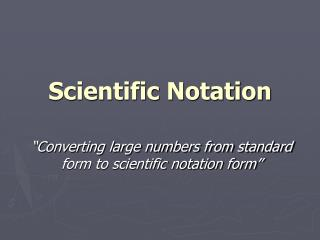DownloadDownload PresentationScientific Notation

# Scientific Notation

Download Presentation## Scientific Notation

- - - - - - - - - - - - - - - - - - - - - - - - - - - E N D - - - - - - - - - - - - - - - - - - - - - - - - - - -
##### Presentation Transcript

1. Scientific Notation “Converting large numbers from standard form to scientific notation form”

2. What is Scientific Notation • Scientific notation is a way to make large numbers easier to work with. 2,890,000,000 (standard form) notice where the decimal is located, at the end of the number

3. Converting standard form to Sc. No. 2,890,000,000 (standard form) To convert to scientific notation we move the decimal point to the left until it is between the 1st and 2nd number. 289 289 x 10 we still have the zeros left from the original number to represent the zeros we write x 10

4. Converting standard form to Sc. No. 2,890,000,000 (standard form) 2.89 x 109what does the exponent 9 represent We moved the decimal point 9 places to the left Which means we multiply 2.89 x10 nine times

5. Converting Standard Form 523,000 (standard form) 5.23 x 105 (scientific notation) Moved the decimal point to the left 5 places 1,747,000 (standard form) 1.747 x 106 (scientific notation) Moved the decimal point to the left 6 places

6. Converting Decimals 0.0127 (standard form) To convert to scientific notation we move the decimal point to the right until it is between the 1st whole number we come to and 2nd number. 127 x 10-2 (scientific notation) We moved the decimal point to the right 2 places and since we are working with decimals the exponents are now a negative

7. Converting Decimals 0.0000786 (standard form) 7.86 x 10-5 (scientific notation) Moved the decimal point to the right 5 places 0.0000001905 (standard form) 1.905 x 10-7 (scientific notation) Moved the decimal point to the right 5 places

8. Scientific Notation – Let’s Try 1.) 7,921,000,000 1.) 0.046 2.) 686,000,000 2.) 0.00703 3.) 806,000 3.) 0.00353 4.) 3,679,000 4.) 0.000067 5.) 8,936,000,000 5.) 0.00000082

9. Scientific Notation – Let’s Try 1.) 7.921 x 109 1.) 4.6 x 10-2 2.) 6.86 x 1082.) 7.03 x 10-3 3.) 8.06 x 105 3.) 3.53 x 10-3 4.) 3.679 x 106 4.) 6.7 x 10-5 5.) 8.936 x 109 5.) 8.2 x 10-7